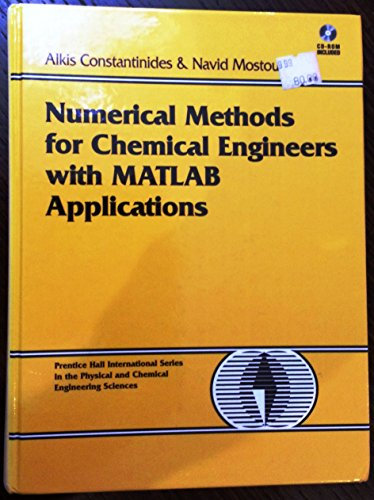# Numerical Methods for Chemical Engineers With Matlab Applications

## Constantinides, Alkis; Mostoufi, NavidVedi tutte le copie di questo ISBN:

Master numerical methods using MATLAB, today's leading software for problem solving.

This complete guide to numerical methods in chemical engineering is the first to take full advantage of MATLAB's powerful calculation environment. Every chapter contains several examples using general MATLAB functions that implement the method and can also be applied to many other problems in the same category.

The authors begin by introducing the solution of nonlinear equations using several standard approaches, including methods of successive substitution and linear interpolation; the Wegstein method, the Newton-Raphson method; the Eigenvalue method; and synthetic division algorithms. With these fundamentals in hand, they move on to simultaneous linear algebraic equations, covering matrix and vector operations; Cramer's rule; Gauss methods; the Jacobi method; and the characteristic-value problem. Additional coverage includes:

Finite difference methods, and interpolation of equally and unequally spaced points

Numerical differentiation and integration, including differentiation by backward, forward, and central finite differences; Newton-Cotes formulas; and the Gauss Quadrature

Two detailed chapters on ordinary and partial differential equations

Linear and nonlinear regression analyses, including least squares, estimated vector of parameters, method of steepest descent, Gauss-Newton method, Marquardt Method, Newton Method, and multiple nonlinear regression

The numerical methods covered here represent virtually all of those commonly used by practicing chemical engineers. The focus on MATLAB enables readers to accomplish more, with less complexity, than was possible with traditional FORTRAN. For those unfamiliar with MATLAB, a brief introduction is provided as an Appendix.

Over 60+ MATLAB examples, methods, and function scripts are covered, and all of them are included on the book's CD

Le informazioni nella sezione "Riassunto" possono far riferimento a edizioni diverse di questo titolo.

L'autore:

ALKIS CONSTANTINIDES is a member of the faculty in the Department of Chemical and Biochemical Engineering at Rutgers, The State University of New Jersey.

NAVID MOSTOUFI is a member of the faculty in the Department of Chemical Engineering, University of Tehran, Iran.

Product Description:

For junior/senior/graduate-level courses in Numerical Methods in departments of Chemical and Biochemical Engineering. Designed for multi-level use, this text explores in detail the derivation of a variety of numerical methods and their application to the solution of engineering problems--with special attention to problems in chemical engineering. It uses MATLAB(R) extensively throughout (for the WINDOWS, Macintosh, and Unix environments)--but focuses clearly on teaching students numerical methods and problem solving skills. It is not a MATLAB manual for simple number-crunching. The companion web site at informit.com/title/9780130138514 contains the MATLAB scripts used throughout the text.

Le informazioni nella sezione "Su questo libro" possono far riferimento a edizioni diverse di questo titolo.

## 1.Numerical Methods for Chemical Engineers with MATLAB Applications

Editore: Prentice Hall (1999)
ISBN 10: 0130138517 ISBN 13: 9780130138514
Nuovo Rilegato Quantit�: 1
Da
GoldenWavesOfBooks
(Fayetteville, TX, U.S.A.)

Descrizione libro Condizione: new. Codice articolo HolzAbe_New_0130138517

Compra nuovo
EUR 93,20
Convertire valuta
Spese di spedizione: EUR 3,33
In U.S.A.
Destinazione, tempi e costi

## 2.Numerical Methods for Chemical Engineers with MATLAB Applications Constantinides, Alkis and Mostoufi, Navid

Editore: Prentice Hall (1999)
ISBN 10: 0130138517 ISBN 13: 9780130138514
Nuovo Rilegato Quantit�: 1
Da
BennettBooksLtd
(San Diego, CA, U.S.A.)

Descrizione libro Condizione: New. New. Codice articolo Q-0130138517

Compra nuovo
EUR 92,45
Convertire valuta
Spese di spedizione: EUR 4,13
In U.S.A.
Destinazione, tempi e costi

## 3.Numerical Methods for Chemical Engineers With Matlab Applications

Editore: Prentice Hall (1999)
ISBN 10: 0130138517 ISBN 13: 9780130138514
Nuovo Rilegato Quantit�: 1
Da
GlassFrogBooks
(Hawthorne, CA, U.S.A.)

Descrizione libro Condizione: New. A+ Customer service! Satisfaction Guaranteed! Book is in NEW condition. Codice articolo 0130138517-2-1

Compra nuovo
EUR 119,87
Convertire valuta
Spese di spedizione: GRATIS
In U.S.A.
Destinazione, tempi e costi